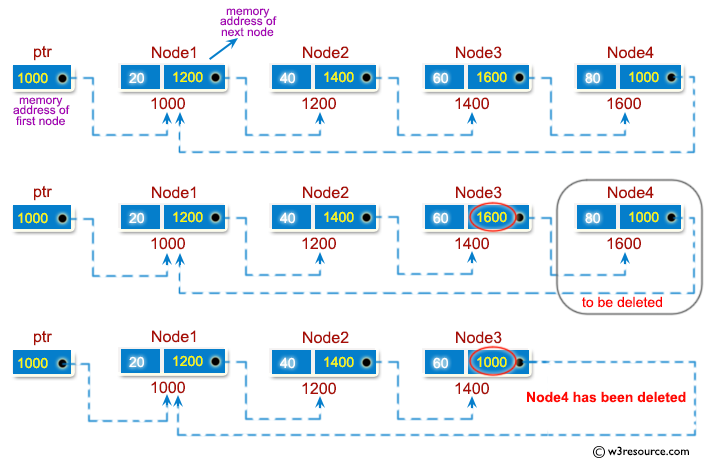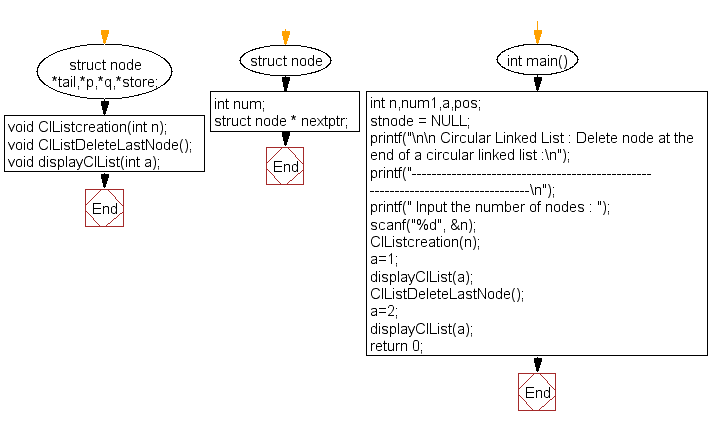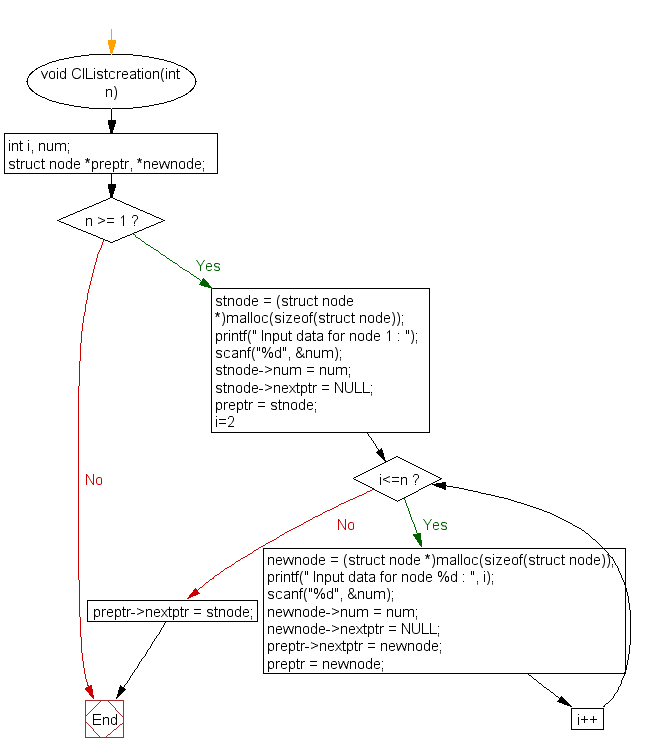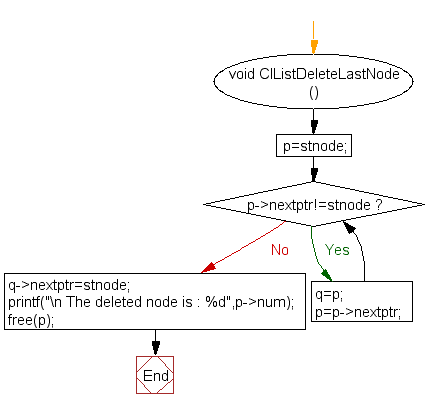﻿ C : Delete the node at the end of a circular linked list

# C Exercises: Delete the node at the end of a circular linked list

## C Doubly Linked List : Exercise-18 with Solution

Write a program in C to delete the node at the end of a circular linked list.

Pictorial Presentation:Sample Solution:

C Code:

``````#include <stdio.h>
#include <stdlib.h>

struct node {
int num;
struct node * nextptr;
}*stnode;

struct node *tail,*p,*q,*store;

void ClListcreation(int n);
void ClListDeleteLastNode();
void displayClList(int a);

int main()
{
int n,num1,a,pos;
stnode = NULL;
printf("\n\n Circular Linked List : Delete node at the end of a circular linked list :\n");
printf("--------------------------------------------------------------------------------\n");

printf(" Input the number of nodes : ");
scanf("%d", &n);
ClListcreation(n);
a=1;
displayClList(a);
ClListDeleteLastNode();
a=2;
displayClList(a);
return 0;
}

void ClListcreation(int n)
{
int i, num;
struct node *preptr, *newnode;

if(n >= 1)
{
stnode = (struct node *)malloc(sizeof(struct node));

printf(" Input data for node 1 : ");
scanf("%d", &num);
stnode->num = num;
stnode->nextptr = NULL;
preptr = stnode;
for(i=2; i<=n; i++)
{
newnode = (struct node *)malloc(sizeof(struct node));
printf(" Input data for node %d : ", i);
scanf("%d", &num);
newnode->num = num;
newnode->nextptr = NULL;	// next address of new node set as NULL
preptr->nextptr = newnode;	// previous node is linking with new node
preptr = newnode;   		// previous node is advanced
}
preptr->nextptr = stnode; 		//last node is linking with first node
}
}

void ClListDeleteLastNode()
{
p=stnode;
while(p->nextptr!=stnode)
{
q=p;
p=p->nextptr;
}
q->nextptr=stnode;
printf("\n The deleted node is : %d",p->num);
free(p);
}

void displayClList(int m)
{
struct node *tmp;
int n = 1;

if(stnode == NULL)
{
printf(" No data found in the List yet.");
}
else
{
tmp = stnode;
if (m==1)
{
printf("\n Data entered in the list are :\n");
}
else
{
printf("\n After deletion the new list are :\n");
}
do {
printf(" Data %d = %d\n", n, tmp->num);

tmp = tmp->nextptr;
n++;
}while(tmp != stnode);
}
}
```
```

Sample Output:

``` Circular Linked List : Delete node at the end of a circular linked list :
--------------------------------------------------------------------------------
Input the number of nodes : 3
Input data for node 1 : 2
Input data for node 2 : 5
Input data for node 3 : 8

Data entered in the list are :
Data 1 = 2
Data 2 = 5
Data 3 = 8

The deleted node is : 8
After deletion the new list are :
Data 1 = 2
Data 2 = 5
```

Flowchart:ClListcreation() :ClListDeleteLastNode() :displayClList() :C Programming Code Editor:

What is the difficulty level of this exercise?

Test your Programming skills with w3resource's quiz.

﻿